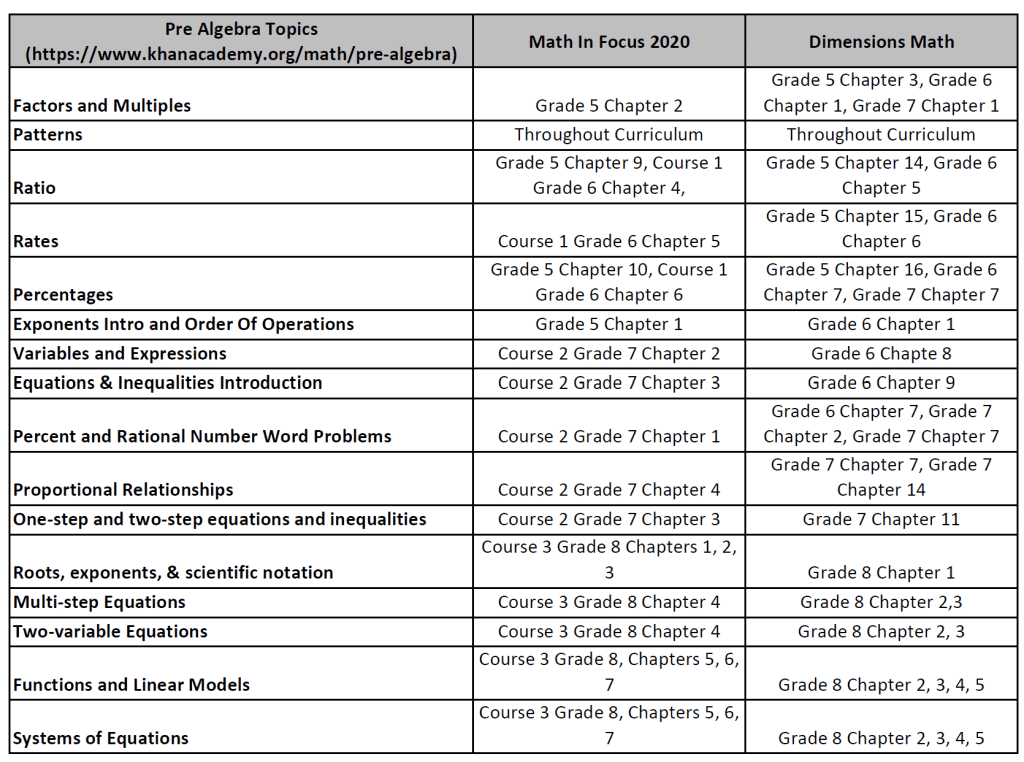Singapore Math Curriculum

# Singapore Math and Pre-Algebra

One common question we get from parents is how the Singapore Math curriculum prepares students for Algebra and what pre-algebra topics are covered in the Singapore Mathematics curriculum. In this article, we will explore the features of the Singapore Math Curriculum, specifically Dimensions Math and Math in Focus 2020. Dimensions Math and Math in Focus are the two curriculums in the United States that provide a curriculum and materials up to Grade 8 currently.

## How the Singapore Math Curriculum Prepares Students For Algebra?

Mathematics is a fundamental subject that plays a crucial role in a student’s academic journey. In recent years, the Singapore math curriculum has gained significant attention and acclaim worldwide for its innovative and effective approach to teaching mathematics. Many parents are curious about how the Singapore math curriculum, prepares students for algebra and pre-algebra.

### Building a Solid Mathematical Foundation:

The Singapore math curriculum places a strong emphasis on building a solid foundation in mathematics. It starts by developing students’ conceptual understanding before introducing abstract mathematical concepts. This approach ensures that students have a deep understanding of mathematical concepts, enabling them to apply their knowledge to problem-solving in pre-algebra and beyond.

### Concrete-Pictorial-Abstract Approach:

One of the hallmarks of the Singapore math curriculum is its Concrete-Pictorial-Abstract (CPA) approach. This method encourages students to move from hands-on, concrete experiences to pictorial representations and then to abstract symbols and equations. By using manipulatives and visual aids, students can visualize mathematical concepts, making them more tangible and easier to comprehend. This visual and experiential learning approach fosters a strong understanding of numbers, operations, and relationships, which are vital when approaching pre-algebra topics.

### Focus on Problem Solving:

Problem-solving is at the core of the Singapore math curriculum. Students are exposed to a wide range of problem-solving strategies and are encouraged to think critically and creatively. The curriculum promotes a problem-solving mindset by presenting real-life and engaging scenarios that require students to apply mathematical concepts to arrive at solutions. This emphasis on problem-solving develops students’ analytical skills and prepares them to tackle the complexities of pre-algebraic equations and algebraic thinking.

### Progressive Learning of Mathematical Topics:

The Singapore math curriculum follows a carefully sequenced and progressive approach to the learning of mathematical topics. It introduces concepts in a logical and coherent manner, allowing students to build on their prior knowledge as they progress through the curriculum. This sequential approach ensures that students have a solid understanding of fundamental mathematical concepts before moving on to more advanced topics, such as pre-algebra. The curriculum gradually introduces algebraic thinking, equations, variables, and functions, laying the groundwork for students to excel in pre-algebra.

### Integration of Multiple Problem-solving Strategies:

The Singapore math curriculum emphasizes the use of multiple problem-solving strategies. Students are exposed to various approaches, including model drawing, bar modeling, logical reasoning, and algebraic manipulation. This diversity of strategies enables students to develop a flexible mindset and choose the most effective approach to solve different types of problems. These problem-solving strategies not only enhance students’ mathematical abilities but also provide them with the tools needed to excel in pre-algebra and algebra, where a similar range of problem-solving techniques is employed.

By emphasizing conceptual understanding, problem-solving skills, and a progressive learning approach, Singapore Math equips students with the necessary foundation to succeed in pre-algebra and beyond. Its Concrete-Pictorial-Abstract approach, focus on problem-solving, and integration of multiple strategies provide students with a strong mathematical toolkit. As a result, students who have undergone the Singapore math curriculum are well-prepared to tackle the challenges of pre-algebra and excel in their mathematical journey.

## Singapore Math Curriculum and Pre-Algebra.

Dimensions Math and Math in Focus are the two curriculums in the United States that provide a curriculum and materials up to Grade 8 currently. In the table below, we have taken the pre-algebra topics listed in Khan Academy and compared them with topics in Dimensions Math and Math in Focus 2020.##Disclaimer: Although every effort has been made to provide complete and accurate information, Singapore Math Learning Center makes no warranties, express or implied, or representations as to the accuracy of the content on this
website. Singapore Math Learning Center assumes no liability or responsibility for any error or omissions in the
information contained in the website or the operation of the website.

## Singapore Math Curriculum For High School

Currently, in the United States, there is no specific Singapore Mathematics curriculum that covers Grades 9 to 12.

While the transition from Singapore math to high school may involve adjustments to new teaching styles or curricula, the underlying skills and mindset fostered by the Singapore math curriculum promote adaptability and love for continual learning. Students who have experienced the Singapore math approach are accustomed to exploring multiple solution strategies, seeking alternative approaches, and embracing challenges. These qualities enable them to adapt to new mathematical concepts and methodologies in high school while maintaining a growth mindset.

Although there is no specific high school curriculum aligned with Singapore math, students who have completed their primary education under this framework are well-prepared for the transition to high school mathematics. The emphasis on conceptual understanding, problem-solving skills, algebraic thinking, and communication abilities equips students with a strong mathematical foundation. With their adaptable mindset and love for continual learning, these students are poised for success in high school mathematics and beyond. The Singapore math curriculum not only prepares students academically but also instills in them a passion for mathematics and the tools necessary to excel in their mathematical journey.

Prices pulled from the Amazon Product Advertising API on:

#### Singapore Math Learning Center

At Singapore Math Learning Center, we provide online tutoring and online courses to those who are using any Singapore Math curriculums or who are interested in the Singapore Math method. For a free math assessment, please contact us.

Disclosure: This post might contain affiliate links and SingaporeMathLearningCenter.Com is a participant in the Amazon Associates program and may earn a small commission from qualifying purchases.